# Perimeter of the park

Find the perimeter of the park in meters in a diamond shape, if the length of its side is 2 km.

x =  8000 m

### Step-by-step explanation:Did you find an error or inaccuracy? Feel free to write us. Thank you!Tips to related online calculators
Do you want to convert length units?

#### You need to know the following knowledge to solve this word math problem:

We encourage you to watch this tutorial video on this math problem:

## Related math problems and questions:

• DiamondThe diamond has a circumference of 48cm. Calculate the length of its side in dm.
• RhombusFind the length of each side of rhombus if the perimeter is 89 cm long.
• Table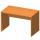Find the circumference of the table, where the long side is 1.28 meters and the short side is 86 cm.
• Fence of the garden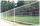The garden has dimensions of 5m and 400cm. How many meters meshes are needed for fencing the plot?
• It is 2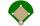It is 90 feet from home plate to first base on a baseball diamond. What is the area of the baseball diamond in square yards?
• Diamond - simple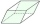Calculate the circumference and area of the diamond if the side is 5 cm long and the height is 3 cm long.
• The diamondThe diamond has an area S = 120 cm2, the ratio of the length of its diagonals is e: f = 5: 12. Find the lengths of the side and the height of this diamond.
• The bookThe book has 280 pages. Each side is a rectangle with sides 15 cm and 22 cm. a) how many leaves has the book? b) at least how many square meters of paper should be used to production this book?
• Rhombus diagonalsIn the rhombus ABCD are given the sizes of diagonals e = 24 cm; f = 10 cm. Calculate the side length of the diamond and the size of the angles, calculate the content of the diamond
• Cups of paintI have a board that is 2 meters wide and 3 meters tall. If one cup of paint cover 1,000 square centimeters. How many cups of paints does I need?
• Central park in city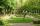The city park has the shape of a rectangle of 180 meters in length and 120 meters in width. People make their walk through the center of the park from one corner to the second. Calculate how many meters this way is shorter than they walked along the path
• TailorTailor has an estimated 11 meters of 80 cm wide fabric. He will shape wholly 7 rectangles measuring 80 x 150 cm and nothing fabric does not remain. How many fabric he have?
• MilimetersHow many millimeters are 1/4 meters?
• RhombusFind the length of the other diagonal and area of the rhombus. The perimeter of a rhombus is 40 cm and one of the diagonals is of length 10 cm.
• Strip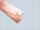From 5.9 cm wide strip should be cut rhombus with area 28 cm2. How long will be its side?
• Rhombus sidesfind the sides of a rhombus, it area is 550 cm square and altitude is 55cm
• Triangle ABCIn a triangle ABC side b measure 10 cm less than the side a and side b is half of the side c. Calculate the length of sides if the circumference of the triangle is 42 cm.